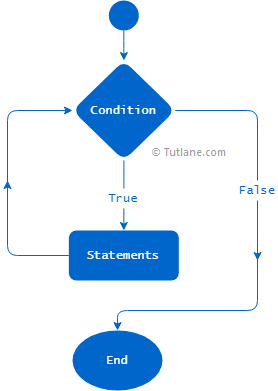# Python While Loop

In python, while loop is useful to execute the block of statements repeatedly until the specified condition is `True`.

Generally, the while loop is useful when you are unsure about the number of times to execute the block of statements. In while loop, you can use the breakcontinue, and pass statements to exit or continue the execution of statements inside the loop based on your requirements.

## While Loop Syntax

In python, we will use the `while` keyword to define the while loop. Following is the syntax of defining while loop in python.

while boolean_expression:
statement1
statement2
…
statementN

If you observe the above while loop syntax, the statements inside of the while loop will repeatedly execute until the specified `boolean_expression` is `True`.

As discussed in python for loop, you need to follow the indentation to create while loop block statements; otherwise, you will get build errors. To know more about specifying the indentation in python, refer to Python basic syntaxes.

## Python While Loop Flow Chart

Following is the flow chart diagram of while loop process flow in python.If you observe the above while loop flow chart diagram, while loop block statements will repeatedly execute until the defined condition is `True`.

## While Loop Example

Following is the example of a while loop in python to execute the block of statements repeatedly until the defined Boolean expression returns `True`.

a = 1
while a < 5:
print(a)
a += 1
print("Outside of while loop")

If you observe the above example, we defined while loop with Boolean expression and followed the required indentation to define the block of statements inside while loop.

When you execute the above python program, you will get the result as shown below.

1
2
3
4
Outside of while loop

## Else Block in While Loop

Same as the python if-else statement, you can also use the `else` block in while loop but the `else` block statements will execute only after completion of while loop execution.

a = 1
while a < 5:
print(a)
a += 1
else:
print("Else block in while loop")

When you execute the above python program, you will get the result as shown below.

1
2
3
4
Else block in while loop

If you observe the above result, the `else` block statements have been executed only after completion of all iterations in a while loop.

## Python Nested While Loop

In python, nested while loops can be created by adding one while loop inside another while loop based on your requirements.

Following is the example of creating the nested while loop in python.

a = 1
while a < 4:
b = 0
while b < 3:
b += 1
print("a:{}, b: {}".format(a,b))
a += 1
print("Outside of Loop")

When you execute the above python program, you will get the result as shown below.

a:1, b: 1
a:1, b: 2
a:1, b: 3
a:2, b: 1
a:2, b: 2
a:2, b: 3
a:3, b: 1
a:3, b: 2
a:3, b: 3
Outside of Loop

## Infinite Loop in Python

In python, you can create an infinite loop using the while loop. In while loop, if the defined condition is always True, the while loop statements will execute continuously as infinite.

Following is the example of creating the infinite loop in python.

a = 1
while a > 0:
a += 1
print("a: {}".format(a))
print("Outside of Loop")

If you observe the above code, the condition (`a > 0`) whatever we defined in while loop will always `True` so, the execution of the while loop will continue as infinite, and the outside of print statement will never execute.

This is how you can use while loop in python to execute the block of statements repeatedly until the defined condition is `True`.